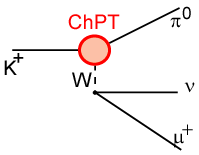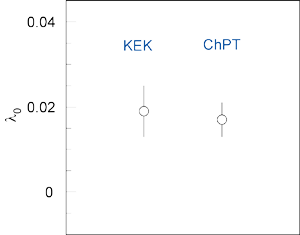## Measurement of Γ(Kμ3)/Γ(Ke3) ratio using stopped positive kaons

### Motivation

 The theory of hadron interaction is quantum chromodynamics (QCD). However, at low energies perturbative methods cannot be applied since the coupling constant becomes very large. One method uses a low energy effective model, Chiral Perturbation Theory (CHPT), which is a rigorous methodology for QCD in the low-energy regions. The predictive power of ChPT can be stringently tested by the form factors of the simplest semileptonic decay Kl3. For the calculation of the Kl3 form factors, a parameterization in terms of the form factors f+ and f0 which are associated with vector and scalar exchange, respectively, are convenient. The form factor f0 is related to f+ and f- throughf0(q2) = f+(q2) + [ q2/(mK2 - mπ2) ]f-(q2) .

The assumption that f+ is linear in q2 and f- is constant leads to f0 linear in q2 as
f0(q2) = f+(0)[ 1 + λ0(q2/mπ2) ] .

The λ+ and λ0 parameters can be predicted in ChPT as,

λ+ = 0.0289±0.0006
λ0 = 0.0168±0.0012 .

Assuming μ-e universality, Kμ3 and Ke3 form factors are identical, the ratio of the Kμ3 and Ke3 decay widths, Γ(Kμ3)/Γ(Ke3), can be related to the λ+ and λ0 parameters as ,

Γ(Kμ3)/Γ(Ke3) = 0.6457 - 0.1531λ+ + 1.5646λ0 .

If the λ+ value derived from Ke3 analysis is used, the λ0 parameter can be determined.

### Results

The Kμ3 and Ke3 events were identified by measuring μ+s and e+s masses, as shown in a figure. The Γ(Kμ3)/Γ(Ke3) ratio can be written as,

Γ(Kμ3)/Γ(Ke3) = N(Kμ3) / N(Ke3) • Ω(Ke3)/Ω(Kμ3) ,

where N and Ω are the number of accepted events and the detector acceptance obtained by a Monte Carlo simulation, respectively. The Γ(Kμ3)/Γ(Ke3) value and the associated λ0 parameter with the μ-e universality assumption were determined to be 

 Γ(Kμ3)/Γ(Ke3) = 0.671 ± 0.007(stat.) ± 0.008(syst.), λ0 = 0.019 ±0.005(stat.) ±0.004(syst.),

which is consistent with the ChPT prediction.### Reference

 J. Gasser and H. Leutwyler, Nucl. Phys. B250, 517 (1985).
 H.W. Fearing, E. Fischbach, and J. Smith, Phys. Rev. D2, 542 (1970); Phys. Rev. Lett. 24, 189 (1970).
 K. Horie et al., Phys. Lett. B513 (2001) 311.